9 Posts

## Subject: Proposal for a new table of scoresYour Tags: Add tags Popular Tags: [View All]
Solitaire dice is according to me one of the hidden gems of Sid Sackson’s “gamut of games”. But I think that the scoring system of this game can be improved. The following review tries to explain why, and how to improve this system.

________________________________________________________________________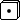COMPUTING PROBABILITIES:

The probabilities for the throws of two dices are given by the following table: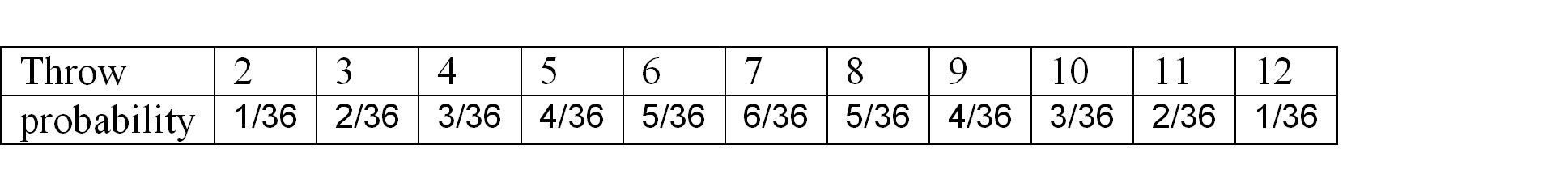Now if you consider that in average a 4 rewards you 60 points three times every thirty-six attempts, this means that your average score for it will be 3/36*60 = 5 points. Similarly, the others expected values are given by: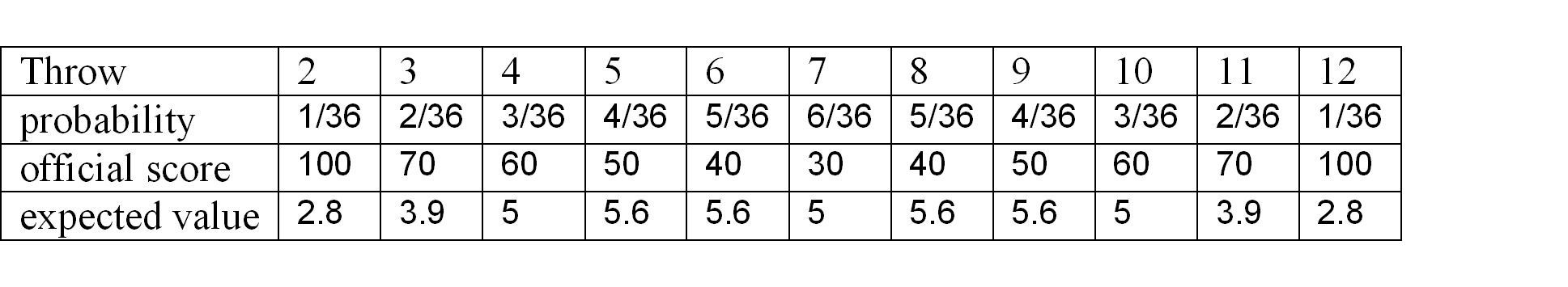It appears that though the “2” and “12” values are given the highest scores, they will usually give you fewer marks than the other throws! In fact, if you try to make a 5, a 6, an 8 or 9, you will probably score twice as much as if you had bet upon a 2 or a 12!

Is this fair to reward to the cautious, and to punish the brave? And is it challenging to play a game with such a biased winning option?
My answer to both questions is “no”, and that’s the reason for…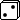A NEW SCORING SHEET:

Here is my suggestion for a balanced scoring sheet: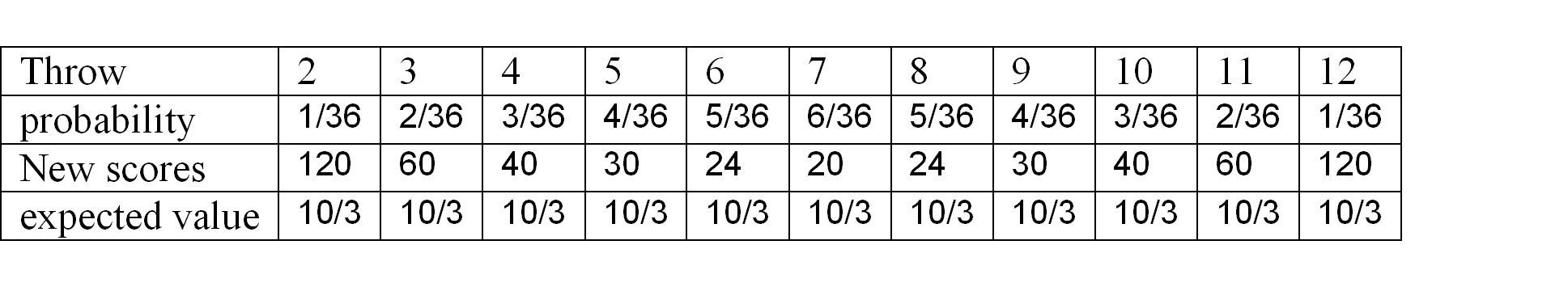The only inconvenient I can see for those scoring values is that they are less easy to remember or to manipulate that the original ones, especially for the “24”. If you consider this as very bothering, then I suggest that you put a 25 in place of the 24: this does not affect much the game’s probabilities, and is quite easier to handle.

Hope you will give it try. Of course any comments or suggestion of yours are very welcomed!
• [+] Dice rolls
I don't see the original game as very bothering. Who says it needs to have an even curve?

That being said... if you go with this... consider changing the -200, say, to -100 or some such.

I would not bother with the 24; heck, I don't like 25, but it's better and simpler.

I have given small thought to changing the 70 (for 3 and 11) to 80.

Thanks for this, JMarc!
• [+] Dice rolls
The new version of this little gem (Extra!) addresses a similar point of view and thus changes the scoring sheet in an apparently interesting way (never played it so far though).

Those of you who have tried the new Extra! Rules, do you think scoring has been improved by the changes?

• [+] Dice rolls
I have played many times Extra! and was shocked of the unbalanced traditional score sheet. You can find the Extra! score sheet here: http://boardgamegeek.com/filepage/88696/extra-score-sheets-p...
• [+] Dice rolls
Markus R wrote:
I have played many times Extra! and was shocked of the unbalanced traditional score sheet. You can find the Extra! score sheet here: http://boardgamegeek.com/filepage/88696/extra-score-sheets-p...
Thanks mate - I've just downloaded the sheet you've contributed to try it out asap!
• [+] Dice rolls
The problem with this analysis is that the probability of each number while rolling 2 dice is NOT the same as rolling 5 dice and splitting them as in this game. It depends on the rejects selected but:

The odds of rolling at least 1 pair that totals 2 while having 1 as a reject are: 546/7776 = 7.06%

The odds of rolling at least 1 pair that totals 2 while NOT having 1 as a reject are: 1526/7776 = 19.62%

Much higher than 1/36 = 2.77%

(Note: 2 & 12 are easy to calculate because you need only look for pairs or better of 1's or 6's. The other numbers are far more difficult because they have multiple ways of forming that total on two dice.)

Basically, 1 is not one of your rejects--you merely need to roll two 1's to get a pair totaling of 2.

If 1 is a reject,
- If you roll neither of your other two rejects, you must roll three or more 1's to be able to form a total of 2
- Otherwise you can select one of the other rejects (or you have a free roll) and you need only roll two 1's.

This analysis assumes you are only trying to form totals of 2--there may strategic reasons for not forming the 2 when you can (like opening another column you don't want to for -200 pts.)
• [+] Dice rolls
Like Richard said, you need to calculate the probability of rolling each number on 2d6 out of 5d6 (7776 possibilities). Ignoring what extra die numbers a player has (and thus would be forced to take one of dice as an extra that is part of the combination), the calculation is not that hard, but I'll leave it for someone better at math to expand on Richard's calculations for 2. I think it will be a shallower curve than that for 36 possibilities, and thus when multiplied by the points for each row, the average scores will be more similar.

You also need to factor in the different probabilities of checking off enough boxes in a row to avoid the penalty. You need to get a 2 four times to avoid the -200 penalty, but six times for a 6, 7, or 8. Needing more boxes for 6-8 numbers should pull down their average relative to 4/5//9/10 and raise the average for 2/3/11/12.

Assuming you roll one of your 3 extra die #'s every turn, but check them off optimally, you will have 22 turns. So if 0.2668 is the throw probability of 2 for (2d6 from 5d6), then perhaps a more accurate average score for 2 whould be:
0.2668 * 100 - 0.2668 * 4/22 * 200 = 16.97
I'm sure that formula is wrong, but it illustrates the point.

• [+] Dice rolls
I used AnyDice to calculate the probability of rolling X with any 2 dice from 5d6. I think these are correct, but I could be wrong.

2: 0.1962 x 100 = 19.62
3: 0.3279 x 70 = 22.95
4: 0.4907 x 60 = 29.44
5: 0.5941 x 50 = 29.71
6: 0.7235 x 40 = 28.94
7: 0.7986 x 30 = 23.96
and repeat for 8-12

I'm still not sure if just multiply by the score gives you the correct average score (since you need a different number of boxes to reach 7x), and I don't know how to factor in the likelihood of not escaping the -200 zone for each number.
• [+] Dice rolls
Ok, this is my best attempt at how to modify the scores as well as alter the number of squares in each row:

2: 0.1962 x 100 = 19.62 (leave as is with 3 squares left/6 squares right)
3: 0.3279 x 60 = 19.67 (leave with 3/6 squares)
4: 0.4907 x 40 = 19.62 (check off 1 square to start with 3/6 squares)
5: 0.5941 x 35 = 20.79 (leave with 4/6 squares)
6: 0.7235 x 30 = 21.71 (leave with 5/6 squares)
7: 0.7986 x 30 = 23.96 (add 1 square to start with 6/6 squares)

• [+] Dice rolls